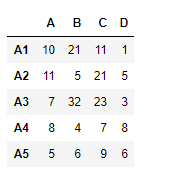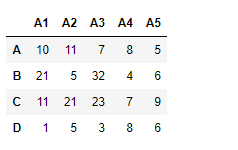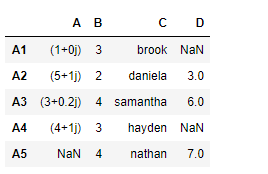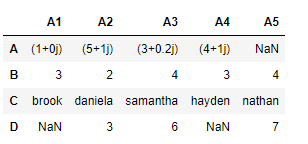# Python | Pandas dataframe.swapaxes()

Python is a great language for doing data analysis, primarily because of the fantastic ecosystem of data-centric python packages. Pandas is one of those packages and makes importing and analyzing data much easier.

Pandas` dataframe.swapaxes()` function interchange axes and swap values axes appropriately. The function takes the name of the axes to be interchanged with as parameter. Based on the axes, it accordingly changes the data as well.

Syntax: DataFrame.swapaxes(axis1, axis2, copy=True)

Parameters :
axis1 : name of the first axes { string}
axis2 : name of the second axes { string}

Returns : y : same as input

Example #1: Use `swapaxes()` function to swap the axes of the dataframe.

 `# importing pandas as pd ` `import` `pandas as pd ` ` `  `# Creating the dataframe  ` `df ``=` `pd.DataFrame({``"A"``:[``10``, ``11``, ``7``, ``8``, ``5``], ` `                   ``"B"``:[``21``, ``5``, ``32``, ``4``, ``6``], ` `                   ``"C"``:[``11``, ``21``, ``23``, ``7``, ``9``], ` `                   ``"D"``:[``1``, ``5``, ``3``, ``8``, ``6``]},  ` `                   ``index ``=``[``"A1"``, ``"A2"``, ``"A3"``, ``"A4"``, ``"A5"``]) ` ` `  `# Print the dataframe ` `df ``# interchange the index and columns axis ` `df.swapaxes(``"index"``, ``"columns"``) `

Output :Example #2: Use `swapaxes()` function to swap the index and column axes with each other. The dataframe has some missing values.

 `# importing pandas as pd ` `import` `pandas as pd ` ` `  `# Creating the dataframe  ` `df ``=` `pd.DataFrame({``"A"``:[``1``, ``5` `+` `1j``, ``3``+``.``2j``, ``4` `+` `1j``, ``None``], ` `                   ``"B"``:[``3``, ``2``, ``4``, ``3``, ``4``], ` `                   ``"C"``:[``"brook"``, ``"daniela"``, ``"samantha"``, ``"hayden"``, ``"nathan"``], ` `                   ``"D"``:[``None``, ``3``, ``6``, ``None``, ``7``]}, ` `                   ``index ``=``[``"A1"``, ``"A2"``, ``"A3"``, ``"A4"``, ``"A5"``]) ` ` `  `# Print the dataframe ` `df ``# interchange the columns and index axis ` ` `  `df.swapaxes(``"index"``, ``"columns"``) `

Output :My Personal Notes arrow_drop_upCheck out this Author's contributed articles.

If you like GeeksforGeeks and would like to contribute, you can also write an article using contribute.geeksforgeeks.org or mail your article to contribute@geeksforgeeks.org. See your article appearing on the GeeksforGeeks main page and help other Geeks.

Please Improve this article if you find anything incorrect by clicking on the "Improve Article" button below.

Article Tags :

Be the First to upvote.

Please write to us at contribute@geeksforgeeks.org to report any issue with the above content.